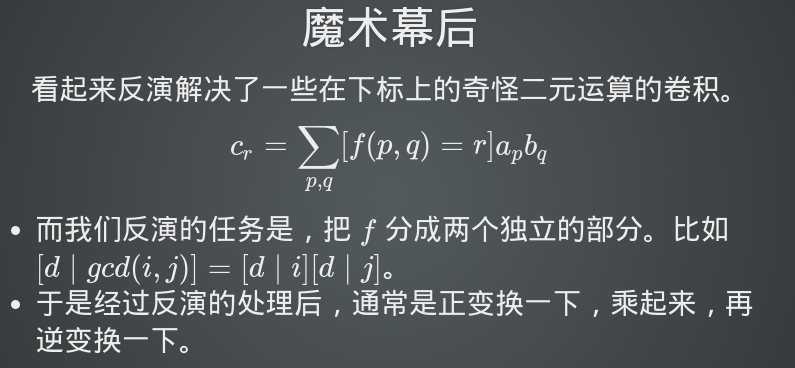# 思维题练习专场-数学篇

### 一，UOJ#62

这道题是反演套反演套反演233333

这题的经验：

首先，反演的过程中不一定要出现$\mu$，我们可以直接用for循环$O(nln_{n})$处理。

所以不能被$\mu$局限住思维，而要观察两个函数之间的相互关系

第二，在遇到一个形如$f(gcd(i,j))$不好化开的时候，我们有这样一种思路：

寻找/构造一个新函数$g(x)$，使得$f(n)=\sum_{d|n}g(d)$

这样的话，我们可以把$f(gcd(i,j))$的限制变成$\sum_{d|i,d|j}g(d)$

这样也许就能找到新的突破口，比如我们可以把$d|i$和$d|j$这两个条件拆开利用，或者改变枚举顺序……之类的

第三，数论和线性代数之间也是有密切联系的。之前一直没有注意到这一点

比如我们的反演过程其实可以表示为乘一个逆矩阵

但是由于莫比乌斯反演的矩阵比较特殊，所以我们能有方便的做法。

此外，此题也有用线性代数理解的方法：我们依然使用上面那个$g$函数，定义下面三个矩阵：

$A_{ij}=[j|i]$，$B_{ij}=[i==j]g(i)$，$C_{ij}=[i|j]$，手推一下可以发现$f=A*B*C$，

这里说一下观察到的一个小性质

如果我们给一个矩阵A乘一个只有对角线上有元素的矩阵B，那么效果相当于给第i行每个

很棒的一点是这三个矩阵的逆都是可以求的，那么我们就获得了$O(n^2)$直接算逆的做法

最后推式子优化也可以做到$O(nlog_{n})$

（不过我个人感觉还是上面三次反演好理解）

*我们具体说一下莫反的线性代数形式

我们知道，$\sum_{d|n} \mu(d) == [n==1]$

那么变一下形，就会有$[m|n] \sum_{d|\frac{n}{m}}\mu(d)==[\frac{n}{m}==1]==[n==m]$

如果我们把$n$和$m$看成下标，上面这个就是一个单位矩阵

接着，我们枚举$c=m*d$，给原式继续变形，则有：

$\sum_{c} [c|n][m|c]\mu(\frac{c}{m})$

现在，我们找两个矩阵A和B，使得$A_{cn}==[c|n]$，$B_{mc}==[m|c]\mu(\frac{c}{m})$

那么会有$BA==I$,这里$I$为单位矩阵

从上面$f$和$g$的定义我们又知道$f=g*A$

两边同乘$B$，那么$f*B=g$，你会发现这也就是莫比乌斯反演的那个式子### 二，loj2513

md傻逼高斯消元有80分部分分？

md那我还n^3个jb啊，这样直接n^2消元就行了

…………可能我与出题人心灵不相通吧……

### 毒瘤之间的交流

$T_{k,i} * T_{l,i} = T_{k#l,i}$

fwt的或/与变换，我们把p|q==r变成p属于r&&q属于r来拆限制，然后用高维前缀和做变换

wq选的题目是一道很不错的博弈论(bzoj4220)

uoj62 zzh

bzoj2627 srs

loj509 yzh

loj6271/548 ryf

uoj328 wxhui

bzoj4220 wq

loj552 ltr

loj2263 wxhao

bzoj4820 wzz

### 最后的总结：

posted @ 2018-04-28 19:47  LadyLex  阅读(509)  评论(0编辑  收藏